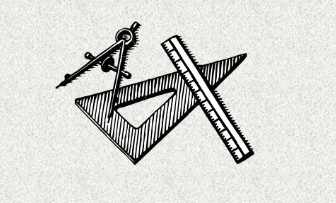# Math Quiz: Bisect Line Segments And Angles.

10 Questions | Total Attempts: 305SettingsUse the following questions to check your understanding of how to construct bisectors of line segments and angles.

• 1.
What does the word BISECT mean?
• A.

To cut something into more than five pieces.

• B.

A shape that has three sides.

• C.

It is a plane with two sets of wings.

• D.

To cut something into two congruent parts or in half.

• 2.
Suppose we wish to construct a perpendicular bisector of line segment EF. We can correctly begin by placing the compass point on E and marking off the distance to which point?
• A.

B

• B.

A

• C.

C

• D.

D

• 3.
Given angle A. The first step in constructing the angle bisector of angle A is to draw  equal arcs that intersect at D from points B and C.
• A.

True

• B.

False

• 4.
Find the value of x.
• A.

X = 47

• B.

X = 33

• C.

X = 19

• D.

X = -47

• 5.
Which diagram shows a correct mathematical construction using only a compass and a straightedge to bisect an angle?
• A.

A

• B.

C

• C.

B

• D.

D

• 6.
Which of the following best describes the construction?
• A.

Ray OC trisects angle BOA.

• B.

Ray OC is parallel to ray OA.

• C.

Ray OC bisects angle BOA.

• D.

Ray OC is parallel to both rays OA and OB.

• 7.
Line segment AB is shown in the diagram. Which two sets of construction marks, labelled I, II, III, and IV, are part of the construction of the perpendicular bisector of line segment AB?
• A.

I and II

• B.

I and III

• C.

II and III

• D.

II and IV

• 8.
Marsha is using a straightedge and compass to do the construction shown. Which best describes the construction Marsha is doing?
• A.

A line through P parallel to line l

• B.

A line through P intersecting line l

• C.

A line through P congruent to line l

• D.

A line through P perpendicular to line l

• 9.
When bisecting an angle, the straightedge is used to
• A.

Mark the point C

• B.

Copy the angle with an arc AB

• C.

Measure the angle BOA

• D.

Connect point C and point O

• 10.
What geometric construction is shown in the diagram below?
• A.

Perpendicular angles

• B.

Perpendicular bisector

• C.

Parallel lines

• D.

Angle bisectorBack to top